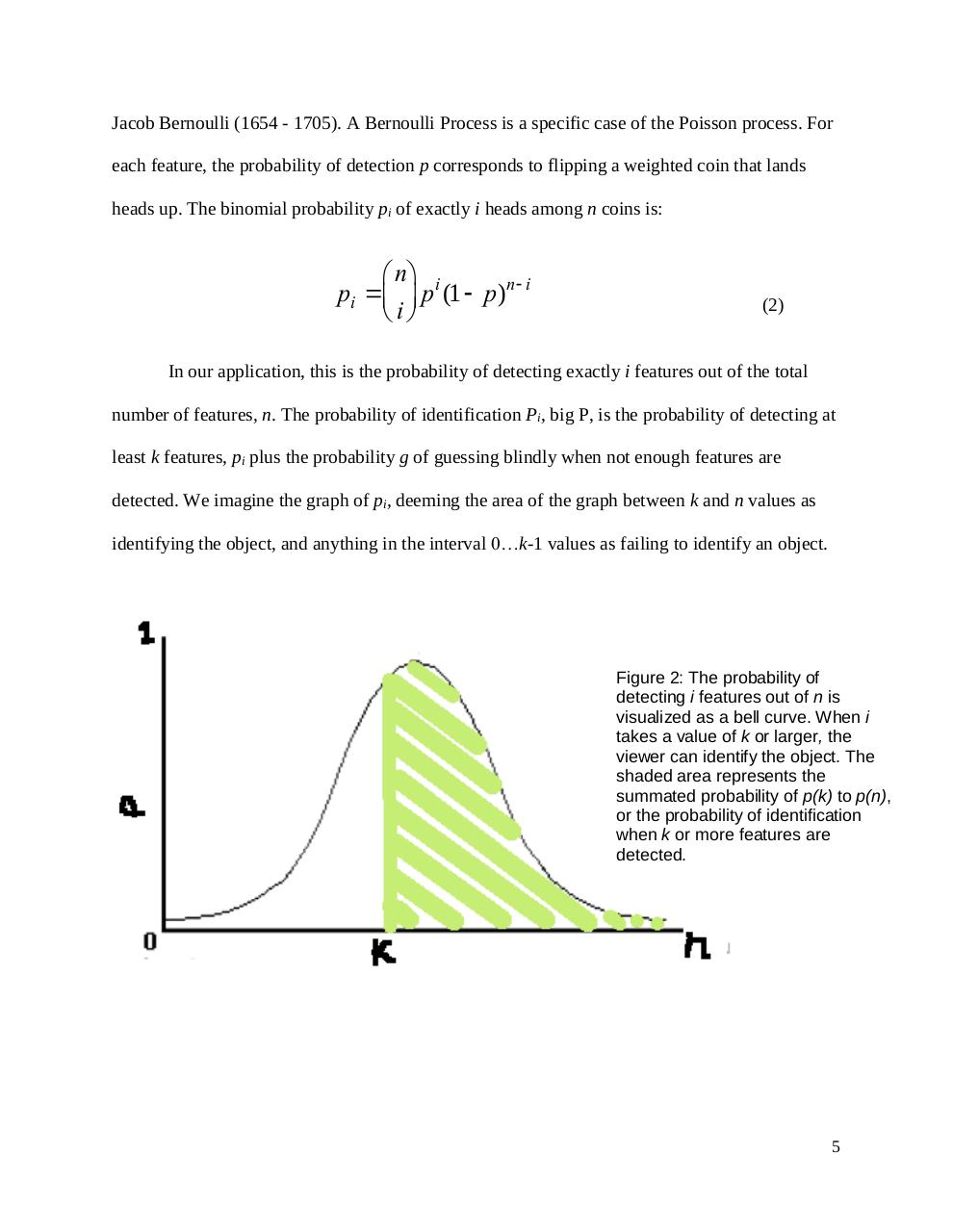Quantifying Visual Feature Detection in Word Identification.pdfPage 1...3 4 56721

Text preview

Jacob Bernoulli (1654 - 1705). A Bernoulli Process is a specific case of the Poisson process. For
each feature, the probability of detection p corresponds to flipping a weighted coin that lands
heads up. The binomial probability pi of exactly i heads among n coins is:

æ nö
pi = ç ÷ p i (1 - p)n-i
è iø

(2)

In our application, this is the probability of detecting exactly i features out of the total
number of features, n. The probability of identification Pi, big P, is the probability of detecting at
least k features, pi plus the probability g of guessing blindly when not enough features are
detected. We imagine the graph of pi, deeming the area of the graph between k and n values as
identifying the object, and anything in the interval 0…k-1 values as failing to identify an object.

Figure 2: The probability of
detecting i features out of n is
visualized as a bell curve. When i
takes a value of k or larger, the
viewer can identify the object. The